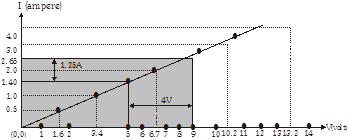3 Tutor System
Starting just at 265/hour

# The values of current I flowing in a given resistor for the corresponding values of potential difference V across the resistor are given below ? V(volts) 1.6 3.4 6.7 10.7 13.2 A(amperes) 0.5 1.0 2.0 3.0 4.0 Plot a graph between V and I and calculate the resistance of that resistor.

Answer The plot between voltage and current is called IV characteristic. The voltage is plotted on x-axis and current is plotted on y-axis. The values of the current for different values of the voltage are shown in the given table.

 V(volts) 1.6 3.4 6.7 10.7 13.2 A(amperes) 0.5 1 2 3 4

The V-I graph is shown below :the value of resistance R is $$R = \frac{V}{I} = \frac{4}{1.25} = 3.2 \Omega$$